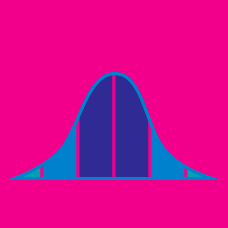Probability

# Covariance - Properties

If the covariances between random variables $X,$ $Y,$ $W,$ and $Z$ are as follows: $\begin{matrix} \text{Cov}(X,Y) = 0.3& \text{Cov}(X,W) = 0.2 \\ \text{Cov}(X,Z) = 0 & \text{Cov}(Y,W) = 0.8 \\ \text{Cov}(Y,Z) = 0 & \text{Cov}(W,Z) = 0.4, \end{matrix}$ what is the covariance between $3X+2W$ and $8Y + 4Z?$

If random variables $X$ and $Y$ have the following variance and covariance: $\text{Var}(X) = 6, \text{Var}(Y) = 8, \text{Cov}(X,Y) = 1,$ what is $\text{Var}( 6X + 8Y )$?

If the covariance between random variables $X$ and $Y$ is $\text{Cov} (X,Y) = 0.3,$ what is $\text{Cov} ( 4X , 2Y )?$

If the covariance between random variables $X$ and $Y$ is $\text{Cov} (X,Y) = 0.2,$ what is $\text{Cov} ( X + 6 , Y + 2 )?$

Let $X$ be a random variable uniformly distributed in the domain $[-1,1],$ and let $Y = X^2.$ What is the covariance between $X$ and $Y?$

×